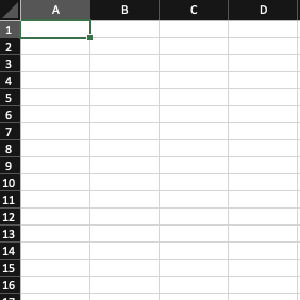## Use a dictionary instead of IF or CASEThe fewer IFs and CASEs the better, right? Every branch of an IF or a CASE is an exception. And we like rules better than exceptions. Here’s one more technique to achieve that.

Consider this code:

``````METHOD get_tax_value_by_code.
IF tax_code = 'VAT23'.
result = 23.
ELSEIF tax_code = 'VAT6'.
result = 6.
ENDIF.
``````

As it is, it’s a pretty innocuous IF. But the day after tomorrow another tax type appears, and then another, and then another. Of course, quickly a CASE would be preferable to the IF. But there is still a better way.

``````TYPES:
BEGIN OF ty_tax,
tax_code TYPE mwskz,
ratio TYPE f,
END OF ty_tax,
ty_taxes TYPE SORTED TABLE OF ty_tax with unique key tax_code.

METHOD get_taxes.
result = VALUE ty_taxes(
( tax_code = 'VAT23' ratio = 23 )
( tax_code = 'VAT6' ratio = 6 ) ).
ENDMETHOD.

METHOD get_tax_value_by_code.
DATA(taxes) = get_taxes( ).
result = taxes[ tax_code = tax_code ].
ENDMETHOD.
``````

• It no longer subdivides into multiple branches as it did with `IF` or `CASE` making it much easier to test;
• You separate the definition of the data from the choice of one of them and so you have a lot more freedom to change the way you define that relationship. You can, for example, very easily choose to define the relationship in a `ZTAX` table:
``````METHOD get_taxes.